## But WHY is the Lattices Bounded Distance Decoding Problem difficult?

This is taken from my Master Thesis on Homomorphic Signatures over Lattices.

### Introduction to lattices and the Bounded Distance Decoding Problem

A lattice is a discrete subgroup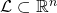, where the word discrete means that each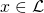has a neighborhood in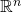that, when intersected with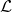results initself only. One can think of lattices as being grids, although the coordinates of the points need not be integer. Indeed, all lattices are isomorphic to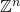, but it may be a grid of points with non-integer coordinates.Another very nice way to define a lattice is: givenindependent vectors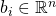, the latticegenerated by that base is the set of all linear combinations of them with integer coefficients: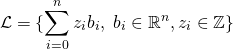Then, we can go on to define the Bounded Distance Decoding problem (BDD), which is used in lattice-based cryptography (more specifically, for example in trapdoor homomorphic encryption) and believed to be hard in general.

Given an arbitrary basis of a lattice, and a pointnot necessarily belonging to, find the point ofthat is closest to. We are also guaranteed thatis very close to one of the lattice points. Notice how we are relying on an arbitrary basis – if we claim to be able to solve the problem, we should be able to do so with any basis.

Now, as the literature goes, this is a problem that is hard in general, but easy if the basis is nice enough. So, for example for encryption, the idea is that we can encode our secret message as a lattice point, and then add to it some small noise (i.e. a small element). This basically generates an instance of the BDD problem, and then the decoding can only be done by someone who holds the good basis for the lattice, while those having a bad basis are going to have a hard time decrypting the ciphertext.

However, albeit of course there is no proof of this (it is a problem believed to be hard), I wanted to get at least some clue on why it should be easy with a nice basis and hard with a bad one (GGH is an example schema that employs techniques based on this).

So now to our real question. But WHY is the Bounded Distance Decoding problem hard (or easy)?

## Why hash tables should use a prime-number size

I read in several books and online pages that hash tables should use a prime number for the size. Nobody really justified this statement properly. Here’s my attempt!

I believe that it just has to do with the fact that computers work with in base 2. Just think at how the same thing works for base 10:

• 8 % 10 = 8
• 18 % 10 = 8
• 87865378 % 10 = 8
• 2387762348 % 10 = 8

It doesn’t matter what the number is: as long as it ends with 8, its modulo 10 will be 8. You could pick a huge power of 10 as modulo operator, such as 10^k (with k > 10, let’s say), but

1. you would need a huge table to store the values
2. the hash function is still pretty stupid: it just trims the number retaining only the first k digits starting from the right.

However, if you pick a different number as modulo operator, such as 12, then things are different:

• 8 % 12 = 8
• 18 % 12 = 6
• 87865378 % 12 = 10
• 2387762348 % 12 = 8

We still have a collision, but the pattern becomes more complicated, and the collision is just due to the fact that 12 is still a small number.

Picking a big enough, non-power-of-two number will make sure the hash function really is a function of all the input bits, rather than a subset of them.